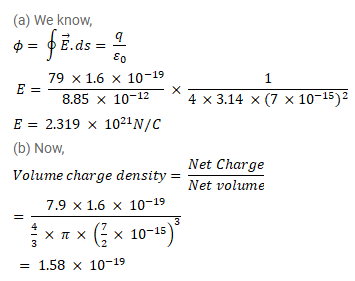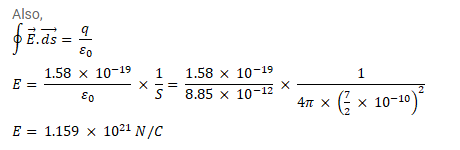# The radius of a gold nucleus (Z = 79) is aboutQuestion:

The radius of a gold nucleus $(\mathrm{Z}=79)$ is about $7.0 \times 10^{-15} \mathrm{~m}$. Assume that the positive charge is distributed uniformly throughout the nuclear volume. Find the strength of the electric field at

(a) the surface of the nucleus and

(b) at the middle point of a radius. Remembering that gold is a conductor, is it justified to assume that the positive charge is uniformly distributed over the entire volume of the nucleus and does not come to the outer surface?

Solution: